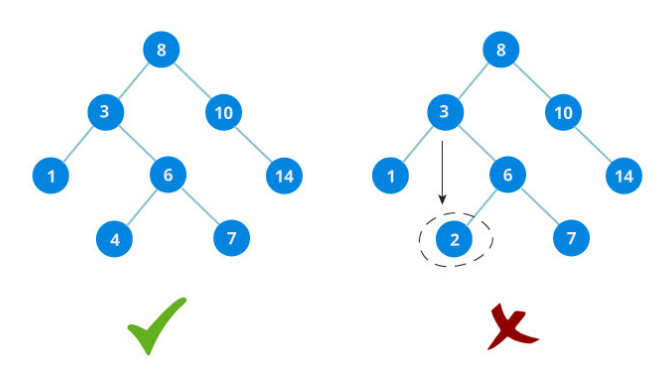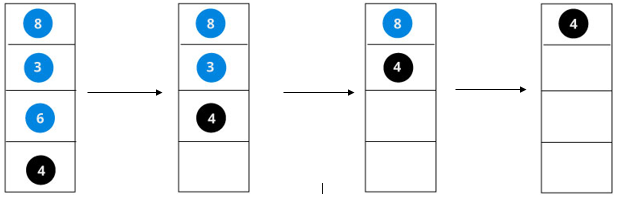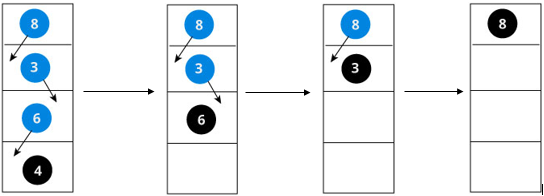# Understand everything about Binary Search Tree

Binary search tree is a data structure that quickly allows us to maintain a sorted list of numbers in a tree data structure.

• It is called a binary tree because has maximuim of two children.
• It is called a search tree because it can be used to search for the presence of a number in `O(log(n))` time.

The properties that separates a binary search tree from a regular binary tree is

1. All nodes of left subtree are less than root node
2. All nodes of right subtree are more than root node
3. Both subtrees of each node are also BST's i.e they have the above two propertiesThe binary tree on the right isn't a binary search tree because the right subtree of the node "3" contains a value smaller that it.

A binary search tree node looks as follows:

``````BSTnode node
{
int data;
BSTnode left_node;
BSTnode right_node;
}
``````

It is the operations following the above restrictions that makes BST special.

There are three basic operations that you can perform on a binary search tree:

• Traversing/ checking if a number is present
• Inserting a number
• Deleting a number

## 1. Check if a number is present in binary search tree

The algorithm depends on the property of BST that if each left subtree has values below root and each right subtree has values above root.

If value is below root, we can say for sure that the value is not in the right subtree; we need to only search in the left subtree and if the value is above root, we can say for sure that the value is not in the left subtree; we need to only search in the right subtree.

## Algorithm

``````    If root==NULL
return NULL;
If number==root->data
return root->data;
If number<root->data
return search(root->left)
If number>root->data
return search(root->right)
``````

Let us try to visualize this with a diagram.

If the value is found, we return the value so that it gets propgated in each recursion step as show in the image below. If you might have noticed , we have called `return search(struct node*)` four times. When we return rither the new node or NULL, the value gets returned again and again unitl `search(root)` returns the final result.## 2. Insert value in Binary Search Tree (BST)

Inserting a value in the correct position is similar to searching because we try to maintain the rule that left subtree is lesser than root and right subtree is larger than root. We keep going to either right subtree or left subtree depending on the value and when we reach a point left or right subtree is null, we put the new node there.

## Algorithm

``````If node == NULL
return createNode(data)
if (data<node->data)
node->left=insert(node->left,data);
else if (data > node->data)
node->right = insert(node->right,data);
return node;
``````

The algorithm isn't as simple as it looks. Let's try to visualize how we add a number to an existing BST.

We have attached the node but we still have to exit from the function without doing any damage to the rest of the tree. This is where the `return node;` at the end comes in handy. In the case of `NULL`, the newly created node is returned and attached to the parent node, otherwise the same node is returned without any change as we go up until we return to the root. This makes sure that as we move back up the tree, the other node connections aren't changes.Implementation:

Implementation of above pseudocode

``````// C program to demonstrate insert operation in binary search tree
#include<stdio.h>
#include<stdlib.h>

struct node
{
int key;
struct node *left, *right;
};

// A utility function to create a new BST node
struct node *newNode(int item)
{
struct node *temp =  (struct node *)malloc(sizeof(struct node));
temp->key = item;
temp->left = temp->right = NULL;
return temp;
}

/* A utility function to insert a new node with given key in BST */
struct node* insert(struct node* node, int key)
{
struct node *newNode(int);
/* If the tree is empty, return a new node */
if (node == NULL) return newNode(key);

/* Otherwise, recur down the tree */
if (key < node->key)
node->left  = insert(node->left, key);
else if (key > node->key)
node->right = insert(node->right, key);

/* return the (unchanged) node pointer */
return node;
}

// Driver Program to test above functions
int main()
{
struct node* insert(struct node* , int);
struct node *root = NULL;
root = insert(root, 8);
insert(root, 3);
insert(root, 10);
insert(root, 1);
insert(root, 6);
insert(root, 4);
insert(root, 7);
insert(root, 5);
insert(root, 10);
insert(root, 9);
insert(root, 13);
insert(root, 11);
insert(root, 14);
insert(root, 12);
insert(root, 2);
return 0;
}
``````

Illustration to insert 2 in above tree:

## 3. Deletion in a binary search tree

Delete function is used to delete the specified node from a binary search tree. However, we must delete a node from a binary search tree in such a way, that the property of binary search tree does'nt violate. There are three situations of deleting a node from binary search tree-

1.The node to be deleted is a leaf node-

In this case we will simply replace the leaf node with the NULL and allocated space will be freed. example-

In the above figure, we are deleting the node 85, since the node is a leaf node, therefore the node will be replaced with NULL and allocated space will be freed.

2.The node to be deleted has only one child-

In this case, replace the node with its child and delete the child node, which now contains the values to be deleted. Simply replace it with the NULL and free the allocated space. example-

In the above figure, the node 12 is to be deleted. It has only one child. The node will be replaced with its child node and the replaced node 12 (which is now leaf node) will simply be deleted.

3.The node to be deleted has two children-

The node which is to be deleted, is replaced with its in-order successor or predecessor recursively uitl the node value(to be deleted) is placed on the leaf of the tree. After the procedure replace the node with NULL and free the allocated space.

example-

In the above figure, the node 50 is to be deleted which is the root node of the tree. The in-order traversal of the tree given below. 6, 25, 30, 50, 52, 60, 70, 75. replace 50 with its in-order successor 52. Now, 50 will be moved to the leaf of the tree, which will simply be deleted.

### Code for insert and search in a BST

``````#include<bits/stdc++.h>
using namespace std;
struct node
{
int key;
struct node *left, *right;
};

// A utility function to search a given node
bool search(struct node* root, int key)
{
// Base Cases: root is null or key is present at root
if (root == NULL || root->key == key)
return true;

// Key is greater than root's key
if (root->key < key)
return search(root->right, key);

// Key is smaller than root's key
return search(root->left, key);
}

// A utility function to create a new BST node
struct node *newNode(int item)
{
struct node *temp =  (struct node *)malloc(sizeof(struct node));
temp->key = item;
temp->left = temp->right = NULL;
return temp;
}

// A utility function to do inorder traversal of BST
void inorder(struct node *root)
{
if (root != NULL)
{
inorder(root->left);
cout<<root->key<<"\n";
inorder(root->right);
}
}

// A utility function to insert a new node with given key in BST
struct node* insert(struct node* node, int key)
{
/* If the tree is empty, return a new node */
if (node == NULL) return newNode(key);

/* Otherwise, recur down the tree */
if (key < node->key)
node->left  = insert(node->left, key);
else if (key > node->key)
node->right = insert(node->right, key);

/* return the (unchanged) node pointer */
return node;
}
void searchKey(Node* &curr, int key, Node* &parent)
{
while (curr != nullptr && curr->data != key)
{
// update parent node as current node
parent = curr;
// if given key is less than the current node, go to left subtree
// else go to right subtree
if (key < curr->data)
curr = curr->left;
else
curr = curr->right;
}
}
// A utility function to delete node from a BST
void deleteNode(Node*& root, int key)
{
// pointer to store parent node of current node
Node* parent = nullptr;
Node* curr = root;
// search key in BST and set its parent pointer
searchKey(curr, key, parent);
if (curr == nullptr)
return;

// Case 1: node to be deleted has no children i.e. it is a leaf node
if (curr->left == nullptr && curr->right == nullptr)
{
// if node to be deleted is not a root node, then set its
// parent left/right child to null
if (curr != root)
{
if (parent->left == curr)
parent->left = nullptr;
else
parent->right = nullptr;
}
// if tree has only root node, delete it and set root to null
else
root = nullptr;
// deallocate the memory
free(curr);	 // or delete curr;
}

// Case 2: node to be deleted has two children
else if (curr->left && curr->right)
{
// find its in-order successor node
Node* successor  = minimumKey(curr->right);
// store successor value
int val = successor->data;
// recursively delete the successor. Note that the successor
// will have at-most one child (right child)
deleteNode(root, successor->data);
// Copy the value of successor to current node
curr->data = val;
}
// Case 3: node to be deleted has only one child
else
{
Node* child = (curr->left)? curr->left: curr->right;
// if node to be deleted is not a root node, then set its parent to its child
if (curr != root)
{
if (curr == parent->left)
parent->left = child;
else
parent->right = child;
}
// if node to be deleted is root node, then set the root to child
else
root = child;
// deallocate the memory
free(curr);
}
}
// Driver Program to test above functions
int main()
{
/* Let us create following BST
50
/     \
30      70
/  \    /  \
20   40  60   80 */
struct node *root = NULL;
root = insert(root, 50);
insert(root, 30);
insert(root, 20);
insert(root, 40);
insert(root, 70);
insert(root, 60);
insert(root, 80);

if(search(root,60)==1)
cout<<"Node Present\n";
else
cout<<"Node not present\n";
// print inoder traversal of the BST
inorder(root);
deleteNode(root,40);
return 0;
}
``````

## Complexity

1. Searching

Worst case time complexity:`O(n)` Average case time complexity:`O(h)`,where h is height of BST.

where N is the number of elements in the Binary Search Tree h = log N for a balanced binary search tree

1. Insertion

Worst case time complexity:`O(n)` Average case time complexity:`O(h)`

1. Deletion

Worst case complexity:`O(n)` Average case time complexity:`O(h)`

1. We can always keep the cost of insert(), delete(), lookup() to O(logN) where N is the number of nodes in the tree - so the benefit really is that lookups can be done in logarithmic time which matters a lot when N is large.
2. We can have ordering of keys stored in the tree. Any time we need to traverse the increasing(or decreasing) order of keys, we just need to do the in-order (and reverse in-order) traversal on the tree.
3. We can implement order statistics with binary search tree - Nth smallest, Nth largest element. This is because it is possible to look at the data structure as a sorted array.
4. We can also do range queries-find keys between N and M (N<=M).
5. BST can also be used in the design of memory allocators to speed up the search of free blocks ( chunks of memory ), and to implement best fit algorithms where we are interested in finding the smallest free chunk with size grater than or equal t osize specifiied in allocation request.

## Applications

1. A Self-Balancing Binary Search Tree is used to maintain sorted stream of data. For example, suppose we are getting online orders placed and we want to maintain the live data (in RAM) in sorted order of prices. For example, suppose we wish to know number of items purchased at cost below a given cost at any moment. Or we wish to know number of items purchased at higher cost than given cost.
2. A Self-Balancing Binary Search Tree is used to implement doubly ended priority queue. With a Binary Heap, we can either implement a priority queue with support of `exctractMin()` or with `extractMax()`. If we wish to support of extractMin() or with extractMax(). If we wish to support both the operations, we use a Self-Balancing Binary Search Tree to do both in `O(Logn)`.
3. There are many more algorithm problems where a Self-Balancing where a Self-Balancing BST is the best suited data structure, like count smaller on right, Smallest Greater on Right Side, etc.

This is a companion discussion topic for the original entry at http://iq.opengenus.org/binary-search-tree/